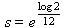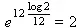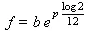On-line GuidesAll GuideseBook StoreiOS / AndroidLinux for BeginnersOffice ProductivityLinux InstallationLinux SecurityLinux UtilitiesLinux VirtualizationLinux KernelSystem/Network AdminProgrammingScripting LanguagesDevelopment ToolsWeb DevelopmentGUI Toolkits/DesktopDatabasesMail SystemsopenSolarisEclipse DocumentationTechotopia.comVirtuatopia.comAnswertopia.comHow To GuidesVirtualizationGeneral System AdminLinux SecurityLinux FilesystemsWeb ServersGraphics & DesktopPC HardwareWindowsProblem SolutionsPrivacy Policy## Chapter 39. Musical Pitches

A musician will call the frequency of a sound its pitch. When the frequencies of two pitches differ by a factor of two, we say they harmonize. This perception of harmony happens because the two sounds reinforce each other completely. Indeed, some people have trouble telling two notes apart when they differ by an octave.

Classical European music divided that perfectly harmonious “factor of two” interval into eight asymetric steps; for this historical reason, it is called an octave. Other cultures divide this same interval into different numbers of steps with different intervals.

More modern European music further subdivides the octave, creating a 12-step system. The modern system has 12 equally spaced intervals, a net simplification over the older 8-step system. The pitches are assigned names using flats (♭) and sharps (♯), leading to each pitch having several names. We'll simplify this system slightly, and use the following 12 names for the pitches within a single octave: A, A#, B, C, C#, D, D#, E, F, F#, G, G#.

The eight undecorated names (A through G and the next A) form our basic octave; the additional notes highlight the interesting asymetries. For example, the interval from A to B is called a whole step or a second, with A♯ being half-way between. The interval from B to C, however is only a half step to begin with. Also, it is common to number the various octaves as though the octaves begin with the C, not the A. So, some musicians consider the basic scale to be C, D, E, F, G, A, B, and the C is in the next higher octave. The higher C is twice the frequency of the lower C.

The tuning of an instrument to play these pitches is called its temperament. A check on the web for reference material on tuning and temperament will reveal some interesting ways to arrive at the tuning of a musical instrument. It is suprising to learn that there are many other ways to arrive at the 12 steps of the scale. This demonstrates that our ear is either remarkably inaccurate or remarkably forgiving of errors in tuning. We'll explore a number of alternate systems for deriving the 12 pitches of a scale. We'll use the simple equal-temperament rules, plus we'll derive the pitches from the overtones we hear, plus a more musical rule called the circle of fifths, as well as a system called Pythagorean Tuning.

Interesting side topics are the questions of how accurate the human ear really is, and can we really hear the differences? Clearly, professional musicians will spend time on ear training to spot fine gradations of pitch. However, even non-musicians have remarkably accurate hearing and are easily bothered by small discrepencies in tuning. The musicians will divide the octave into 1200 cents. Errors on the order of 50 cents, 1/24 of the octave, are noticable even to people who claim they are “tone deaf”. When two tunings produce pitches with a ratio larger than 1.0293, it is easily recognized as out of tune.

These exercises will make extensive use of loops and the list data structure.

## Equal Temperament

The equal temperament tuning divides the octave into twelve equally sized steps. Moving up the scale is done by multiplying the base frequency by some amount between 1 and 2. If we multiply a base frequency by 2 or more, we have jumped to another octave. If we multiply a base frequency by a value between 0 and 0.5, we have jumped into a lower octave. When we multiply a frequency by values between 0.5 and 1, we are computing lower pitches in the same octave. Similarly, multiplying a frequency by values between 1 and 2 computes a higher pitch in the same octave.

We want to divide the octave into twelve steps: when we do a sequence of twelve multiplies by this step, we should arrive at an exact doubling of the base frequency. The steps of the octave, then, would be b , b × s , b × s × s , ... up to b × s 12 = 2× b . This step value, therefore is the following value.If we multiply this 12 times for each of the 12 steps, we find the following.For a given pitch, p , from 0 to 88, the following formula gives us the frequency. We can plug in a base frequency, b of 27.5 Hz for the low A on a piano and get the individual pitches for each of the 88 keys.

Equation 39.1. Musical PitchesEqual Temperament Pitches. Develop a loop to generate these pitches and their names. If you create a simple tuple of the twelve names shown above (from "A" to "G#"), you can pick out the proper name from the tuple for a given step, `s`, using `int( s % 12 )`.

Check Your Results. You should find that an "A" has a pitch of 440, and the "G" ten steps above it will be 783.99 Hz. This 440 Hz "A" is the most widely used reference pitch for tuning musical instruments.Published under the terms of the Open Publication License Design by Interspire